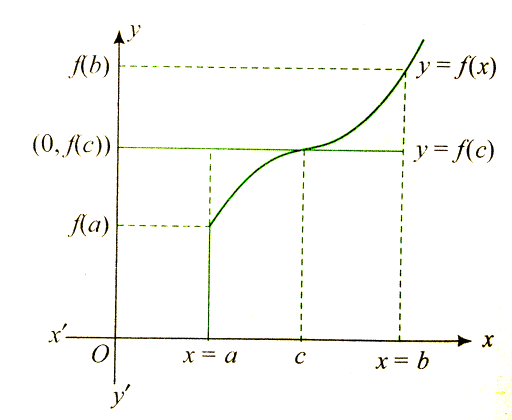If y=f(x) is a monotonic function in (a,b), then the area bounded | FiloClass 12

Math

Calculus

Area548

If y=f(x) is a monotonic function in (a,b), then the area bounded by the ordinates at .Differentiating w.r.t. c, we get

For maxima and minima ,

Hence,

Hence, A is minimum when .
If the area enclosed by between two consecutive points of extremum is minimum, then the value of a is548Connecting you to a tutor in 60 seconds.